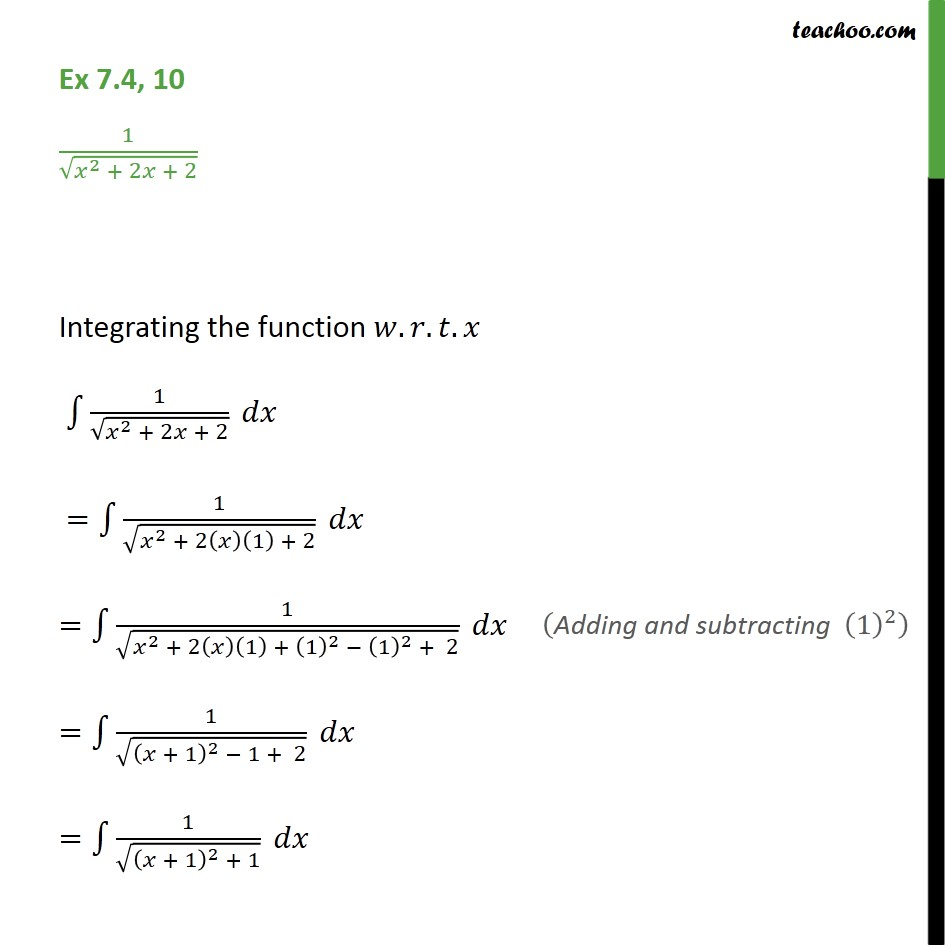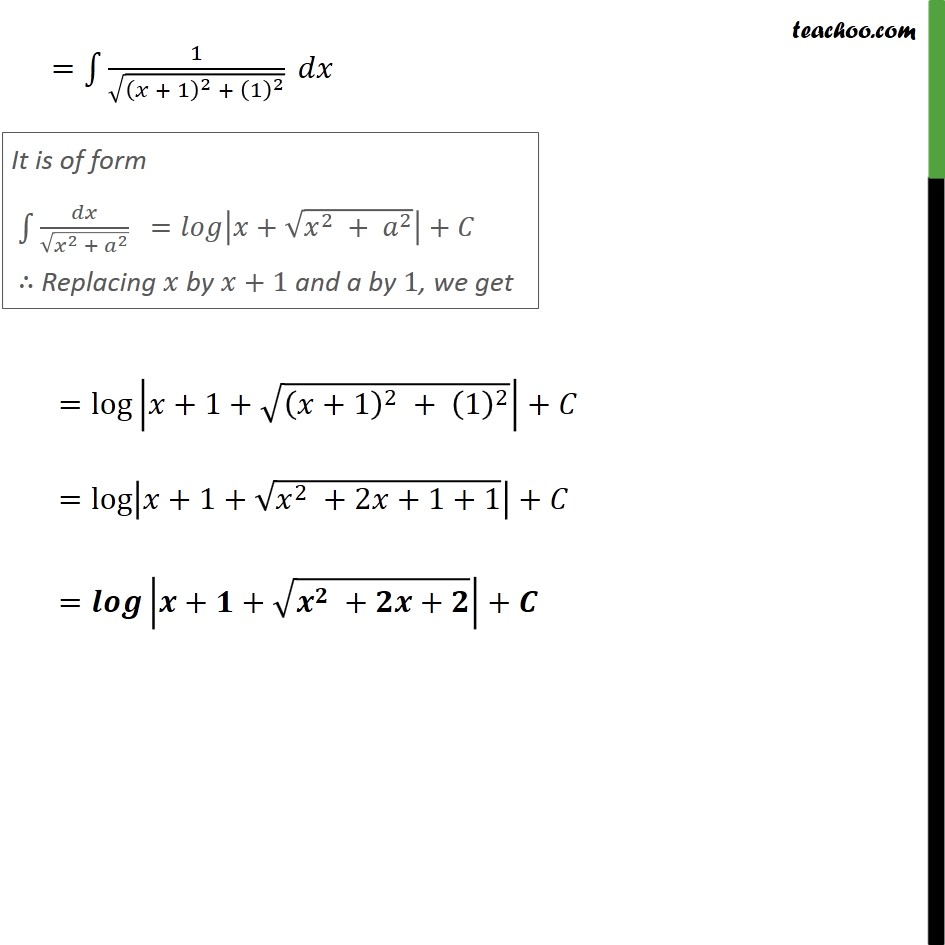1. Chapter 7 Class 12 Integrals (Term 2)
2. Serial order wise
3. Ex 7.4

Transcript

Ex 7.4, 10 1/ ( ^2 + 2 + 2) Integrating the function . . . 1 1/ ( ^2 + 2 + 2) = 1 1/ ( ^2 + 2( )(1) + 2) = 1 1/ ( ^2 + 2( )(1) + (1)^2 (1)^2 + 2) = 1 1/ (( + 1)^2 1 + 2) = 1 1/ (( + 1)^2 + 1) = 1 1/ (( + 1)^2 + (1)^2 ) =log | +1+ (( +1)^2 + (1)^2 )|+ =log | +1+ ( ^2 +2 +1+1)|+ = | + + ( ^ + + )|+

Ex 7.4